# Causal interpretation of the hazard ratio from RCTs when proportional hazards holds

In 2015 I wrote a post about the causal interpretation of hazard ratios estimated in randomised trials, following a paper by Aalen and colleagues. One of the arguments made in that paper was that the hazard ratio does not have a valid interpretation as a causal effect in this setting, even when the proportional hazards assumption holds:

This makes it unclear what the hazard ratio computed for a randomized survival study really means. Note, that this has nothing to do with the fit of the Cox model. The model may fit perfectly in the marginal case with X as the only covariate, but the present problem remains.

With recent discussions on estimands in light of the estimand addendum to ICH E9, I have been thinking more on the argument/claim by Aalen et al.

Suppose we have a randomised trial where we know (somehow) that the ratio of the hazards in the two groups is constant over time – that is the proportional hazards assumption holds. Suppose that the true hazard ratio comparing treatment 1 to treatment 0 is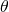. Then due to the relationship between the survival function and hazard, as is well known, it follows that: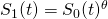where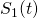and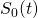are the marginal survival functions in the two treatment groups. This can then be re-expressed as: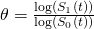Thus under proportional hazards, at any time, the hazard ratio is equal to the ratio of the log of the survival probabilities under the two treatments to this time. The survival functions can be expressed as the population means of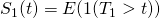where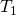denotes the potential failure time under treatment 1 for a randomly selected individual from the population (and similarly for treatment 0). Thus the hazard ratio can be expressed as a functional of the potential outcomes under the two treatments.

Admittedly the interpretation is not that nice, but I would argue the hazard ratio nevertheless does have a causal interpretation (assuming proportional hazards holds). If at a particular time t the survival probabilities are both close to 1, the preceding expression can be approximated to show the hazard ratio is approximately the relative risk of failure under the two treatments, something which has also been written about before.

I am certainly not a causal inference expert, so I do not know what is required to claim that a quantity is a valid causal effect measure. But it would seem to me that if one views the hazard ratio as the previously described ratio of (logged) marginal probabilities, at least when proportional hazards holds, it is a valid causal effect measure. If any causal inference or survival analysis people can help, or point out that I am missing something obvious, please add a comment!

### 7 thoughts on “Causal interpretation of the hazard ratio from RCTs when proportional hazards holds”

1.This is exactly Stijn Vansteelandt’s opinion on this, I believe!

•Thank you Rhian!

2.Odd Aalen replied via email to the post:

Thanks, Jonathan, for comments on our paper. You define a hazard ratio based on comparing two potential survival times and assume the hazard ratio is constant. My response would be that this hazard ratio is not a treatment effect. There will almost always be unmeasured frailty effects. Typically this will mean that the surviving members of the group with the best treatment will get a different mix of frailties when time is running compared with the group with inferior treatment. So your hazard ratio will measure the direct effect of treatment for an individual plus an effect coming from a different mix in the two groups. Initial randomization does not change this issue.

So the hazard ratio is not a pure biological effect of treatment. It is also dependent on the heterogeneity of the treatment groups, and on the extent to which one can adjust for various factors. Typically, this will result in an underestimation of the real treatment effect.

This is closely related to the issue of survival collider bias which is an important concern in epidemiology and survival analysis. In a paper from our group we study this issue both from a frailty point of view and also using causal DAGs. We show how well known paradoxes like false protectivity and the obesity paradox can be understood in terms of frailty models. Reference: Stensrud, M. J., Valberg, M., Røysland, K., & Aalen, O. O. (2017). Exploring selection bias by causal frailty models: the magnitude matters. Epidemiology, 28(3), 379-386.

Still, of course, the Cox model is very important and useful. No method is perfect. What we are doing is to take a hard look at what the results mean, and then it is not so clear as people might think. This is important because the method is used in thousands of clinical trials, for instance, and so it has a tremendous impact. Therefore one should understand the shortcoming of the results as well. It should be noticed also that the assumption of proportional hazards is often not fulfilled. This is seen even in top clinical journals, without any comments being made on the lack of proportionality. So there is a world of statistics where we discuss the principles, and a world out there where they apply our methods to large issues without too much insight into the basis of the methods. We should be concerned about that one as well.

•First, thank you Odd for taking the time to reply. I should have been clearer in my post. I wholeheartedly agree with the article in regards the effects of frailty and that the hazard ratio, even in an RCT, cannot be legitimately interpreted as a causal effect on the hazard of an individual. Rather my intention was to ask: under proportional hazards, is the number corresponding to the hazard ratio related to something which is a valid causal quantity? As described in the post, I think it is equal to the ratio of the log survival probabilities under the two treatments to any time t. For small t (low risk), this quantity is approximately equal to the risk ratio, where the risk is the risk of failure within time t. For larger t (higher risks) this is not the case, and the interpretation is not very nice. However, this number (equal to the hazard ratio) is still arguably a valid causal effect measure, since it is a contrast of two functionals of the potential outcomes/failure times under the two interventions under consideration.

3.An additional point: under the stated conditions, the hazard ratio (obviously?) has the causal interpretation that if we assign the population to receive the new intervention, the marginal/population hazard will at all times be theta times what the marginal/population hazard would have been had the population instead been assigned to receive the control treatment. This may therefore be of relevance for policy level decisions.

4.I think I agree with both Odd and Jonathan. I think, with Odd, that in practice there is a problem. We are never justified in assuming that we have identified everything. We can always believe in hidden causes. My personal (inexpert view) is that this is a general problem with single parameter models (that is to say, dependent on a predictor only). Another example is Poisson regression. (This is not to say that all two parameter models escape this.)
A practical consequence is that it is usually dangerous to assume that simple causal models apply when faced with rich data sets. Pure Poisson regression is always indefensible
However, I would also maintain that the reverse is the case. Naive analysis of survival data using dichotomies have led analysts to exaggerate the frailty element. For this purpose I think it can be useful, purely as an illustration, to show what would happen if the extreme counterfactual model applied. Of course, this is merely a stalking horse that nobody believes in, but it can be useful to issue a warning shot that the magnitude of a frailty component cannot be assessed naively. An example is given here https://errorstatistics.com/2016/08/02/s-senn-painful-dichotomies-guest-post/

•